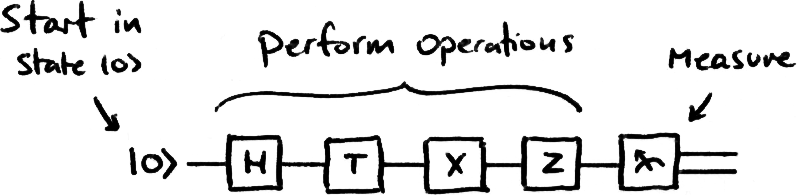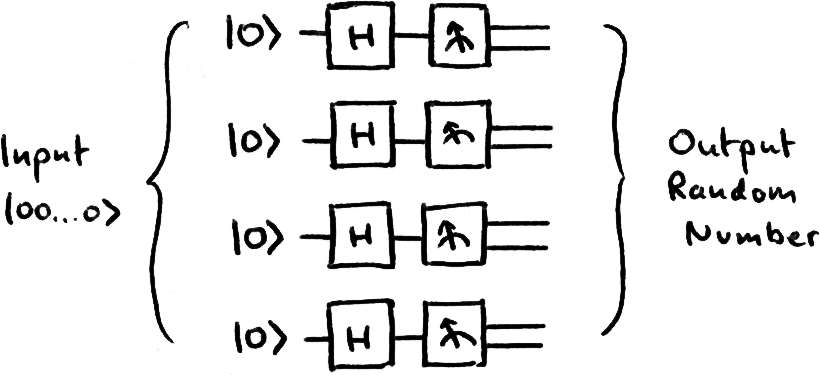# Quantum Computing

## Quantum Circuits

In this section we introduce quantum circuits, a common notation used for communicating quantum algorithms.

## Quantum Circuits

We normally start with our qubits in the state |0⟩, and perform a sequence of operations on them. We use quantum circuits to show this: each line represents a qubit, each box represents an operation and the operations are carried out in chronological order from left to right.## Example Algorithm: Random Number Generator

We now know enough to create our first quantum algorithm! We start with possibly the most simple algorithm we can think of on a quantum computer- a true random number generator.

#### Algorithm: